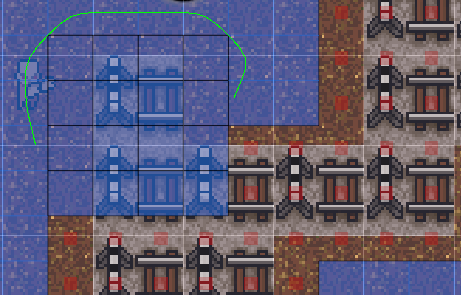# 2D Runtime Walkable Node

Hello,

I’m trying to make Node Walkable at Runtime, it turns blue on the Scene View, but with black outline. So agent can’t walk through this nodes. How can i solve this?

Image:Code:

``````	public class Tester : MonoBehaviour
{
private AstarPath _astar = null;
private GridGraph _graph = null;

private void Start()
{
_astar = AstarPath.active;
_graph = _astar.data.gridGraph;
}

private void Update()
{
if (Input.GetMouseButtonDown(1))
{
Vector2 mousePosition = MousePosition.WorldValue;
int x = (int)mousePosition.x;
int y = (int)mousePosition.y;

{
GraphNode node = _graph.GetNearest(mousePosition).node;
node.Walkable = true;
_graph.CalculateConnectionsForCellAndNeighbours(x, y);
}));
}
}
}
``````

Hi

You need to pass the node’s X and Z coordinates in the grid, not the world coordinates.
Use

``````GridNode node = graph.GetNearest(mousePosition).node as GridNode;
node.Walkable = true;
graph.CalculateConnectionsForCellAndNeighbours(node.XCoordinateInGrid, node.ZCoordinateInGrid);
``````